﻿ Example of Internal/External Pressure Design – Oil & Gas Industry Technology Updates

## Example of Internal/External Pressure Design

Problem
Determine the minimum required thickness of a cylindrical shell and hemispherical heads of a welded pressure vessel designed for an internal pressure of 100 psi at a design temperature of 250°F. There is no corrosion. The shell, which contains a longitudinal butt weld, is also butt welded to seamless heads. All Category A butt joints are Type (1) with full radiography (RT). E = 1.00 for all calculations. The shell has a 5 foot 0 inch inside radius and is 30
foot 0 inch long from tangent to tangent.

Also determine the minimum required thicknesses of the same vessel designed for an external pressure of 15 psi at 100°F without stiffening rings. What is the stiffening ring spacing if the required thickness of internal pressure is used?

Solution
1. For SA 515 Gr. 60, the allowable tensile stress from Table UCS-23 at 100°F is 15.0 ksi, and the external pressure chart is Figure 5-UCS-28.2.
2. As is generally the case for internal pressure on a cylinder, when E = 1.00 for all butt joints, UG-27(c)(1) for circumferential stress (hoop stress) controls over UG-27(c)(2) longitudinal stress by: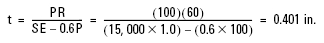Check for applicability of using UG-27(c)(1):
Is t < R/2? 0.400 in. < 30 o.k.
Is P < 0.385 SE? 100< 0.385 (15,000)(1) = 5,775 o.k.
3. For internal pressure on hemispherical heads, use UG-32(f):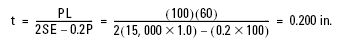Is t < 0.365 L? 0.200 < 0.365 (60) = 21.9 o.k.

Is P < 0.665 SE? 100 < 0.665 (15,000)(1) = 9,975 o.k.
4. For external pressure on cylinder, use UG-28 and Appendix 5:
For cylindrical shells with formed heads on the end the length of the shell plus 1/3 of the depth of each head is used to determine the effective lengths (L) (see UG-28).
Determine the effective length without stiffening rings = 1/3 of each head depth plus straight length =
(1/3)(2)(60) + 360 = 400 in.
Assume tmin. for internal pressure of 0.400 in. and Do = 120 + 2(0.4)
L/Do = 400/120.8 = 3.31
Do/t = 120.4/0.4 = 301
a. Enter Figure 5-UGO-28.0 with L/Do= 3.31 and read across to sloping line of Do/t= 301. Read A = 0.000075
b. Enter Figure 5-UCS-28.2 with A = 0.000075 and the modulus of elasticity E = 29.0 ´ 106 which is off the left
Following Step (7) of UG-28(c):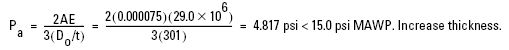Assume t = 5/8 in. = 0.625 in. and Do = 120 + 2(0.625) = 121.25 in.
L/Do = 400/121.25 = 3.30; Do/t = 121.25/0.625 = 194
a. From Figure 5-UGO-28.0, A = 0.00014
b. Recalculate Pa: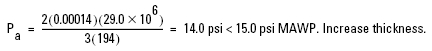Assume t = 11/16 in. = 0.6875 in. and Do = 120 + 2(0.6875) = 121.375 in.
L/Do = 400/121.375 = 3.30
Do/t = 121.375/0.6875 = 177
A = 0.00017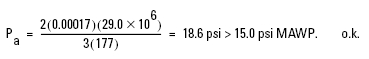Further calculations show that tmin. = 0.64 in. for 15.0 psi external pressure.
5. For external pressure on hemispherical head, use UG-33(c), UG-28(d), and Appendix 5.
First assumption, use tmin. for internal pressure of t = 0.200.
Assume t = 0.200 in. and Ro = 0.5(120 + 2 ´ 0.2) = 60.2 in.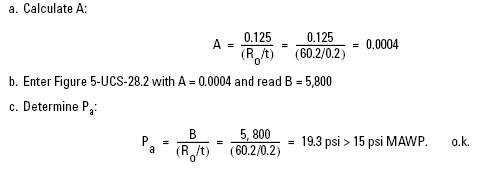Of interest is the fact that for 100 psi internal pressure the minimum required thickness of the cylinder is 0.401, while for 15.0 psi external pressure the minimum required thickness is 0.636 in. For the head, the minimum required thickness is only 0.200 in. for internal pressure, while for external pressure the minimum required thickness is less than 0.200 inches.

If a thickness between 0.400 in. and 0.636 in. is desired for the cylinder, stiffening rings are required on the cylinder to obtain a smaller value of L to use in the calculation of Pa. By “trial-and-error,” the approximate maximum stiffening ring spacing with the minimum thickness required for internal pressure of 0.400 is 120 in. as follows:
Assume t = 0.400 in. and L = 120 in.
L/Do = 120/120.8 = 0.993
Do/t = 302
a. Enter Figure 5-UCS – 28.0 and A = 0.00025
b. Enter Figure 5-UCS – 28.2 and B = 3500
c. Determine Pa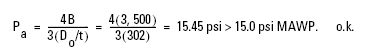This indicates that the optimum design would be one where the shell was thickened above 0.400 in. with stiffening rings being placed at a spacing larger than 120 in. center-to-center. The optimum design would be obtained by “trial-and-error.” After the “best” thickness and stiffening ring spacing is determined, the design of the stiffening ring is developed according to UG-29.